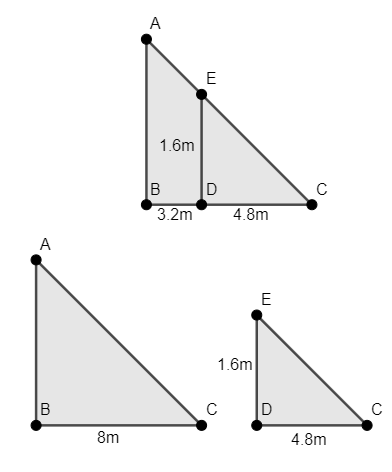QuestionAnswers

# A 1.6 m tall girl stands at a distance of 3.2 m from a lamppost and casts a shadow of 4.8 m on the ground. Find the height of the height of the lamppost by using(1) Trigonometric ratio(2) Property of similar triangles.Verified
129.9k+ views
Hint: Draw the angle of elevations at two different points and apply $\tan \theta$ to the two right angled triangles and we will get two equations and then we have to compute the height of the tower.Considering the height of the tower as AB.
Using Trigonometric ratios:
We know the trigonometric ratio $\tan \theta$ is given as $\tan \theta =\dfrac{P}{B}$, where P is the perpendicular of the triangle and B is the base.
In $\Delta CDE$
$\tan \theta =\dfrac{DE}{DC}$
$\tan \theta =\dfrac{1.6}{4.8}$
$\tan \theta =\dfrac{1}{3}$
In $\Delta CBA$
$\tan \theta =\dfrac{AB}{BC}$
We know $\tan \theta =\dfrac{1}{3}$, substituting the value of $\tan \theta$ in the expression above
$\dfrac{1}{3}=\dfrac{AB}{3.2+4.8}$
Simplifying the expression above we get,
$\dfrac{1}{3}=\dfrac{AB}{8}$
By cross multiplying the terms we get the value of AB as,
$AB=\dfrac{8}{3}$
Dividing 8 by 3 to get the value as a decimal,
The height of the pole is 2.67m.

Using property of similar triangles:

In $\Delta CDE$and $\Delta CBA$
We know the both the angles $\angle CDE$ and $\angle CBA$ are of $90{}^\circ$ measure therefore,
$\angle CDE=\angle CBA$
We know the both the angles $\angle DCE$ and $\angle BCA$ are common in both the triangles,
$\angle DCE=\angle BCA$
By (angle-angle) AA similarity criteria
$\Delta CDE\sim \Delta CBA$
Now we know the ratio of sides of two similar triangles is equal,
Expressing the above statement mathematically we get,
$\dfrac{DE}{AB}=\dfrac{CD}{BC}$
Substituting the values of DE, CD, BC we get,
$\dfrac{1.6}{AB}=\dfrac{4.8}{8}$
Cross multiplying the terms we get AB as,
$AB=\dfrac{1.6\times 8}{4.8}$
$AB=\dfrac{8}{3}$
AB = 2.67m
Hence found by using both trigonometric ratio and property of similar triangles.

Note: the angle of elevation is the angle between the horizontal line from the observer and the line of sight to an object that is above the horizontal line. As the person moves from one point to another angle of elevation varies. If we move closer to the object the angle of elevation increases and vice versa.